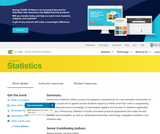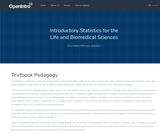# Mathematics

<!--td {border: 1px solid #ccc;}br {mso-data-placement:same-cell;}-->Second-order subjects in Mathematics include Algebra, Calculus, Functions, Geometry, Numbers and Operations, Measurement and Data, Ratios and Proportions, Statistics and Probability, and Trigonometry.

2 affiliated resources

# Search Resources

View
Selected filters:Unrestricted Use
CC BY
Rating

Introductory Statistics follows scope and sequence requirements of a one-semester introduction to statistics course and is geared toward students majoring in fields other than math or engineering. The text assumes some knowledge of intermediate algebra and focuses on statistics application over theory. Introductory Statistics includes innovative practical applications that make the text relevant and accessible, as well as collaborative exercises, technology integration problems, and statistics labs.

Subject:
Statistics and Probability
Material Type:
Textbook
Provider:
Rice University
Provider Set:
OpenStax College
Author:
Barbara Ilowsky
Susan Dean
07/19/2013Conditional Remix & Share Permitted
CC BY-SA
Rating

Introduction to Statistics for the Life and Biomedical Sciences has been written to be used in conjunction with a set of self-paced learning labs. These labs guide students through learning how to apply statistical ideas and concepts discussed in the text with the R computing language.

The text discusses the important ideas used to support an interpretation (such as the notion of a confidence interval), rather than the process of generating such material from data (such as computing a confidence interval for a particular subset of individuals in a study). This allows students whose main focus is understanding statistical concepts to not be distracted by the details of a particular software package. In our experience, however, we have found that many students enter a research setting after only a single course in statistics. These students benefit from a practical introduction to data analysis that incorporates the use of a statistical computing language.

In a classroom setting, we have found it beneficial for students to start working through the labs after having been exposed to the corresponding material in the text, either from self-reading or through an instructor presenting the main ideas. The labs are organized by chapter, and each lab corresponds to a particular section or set of sections in the text.

There are traditional exercises at the end of each chapter that does not require the use of computing. More complicated methods, such as multiple regression, do not lend themselves to hand calculation and computing is necessary for gaining practical experience with these methods. The lab exercises for these later chapters become an increasingly important part of mastering the material.

An essential component of the learning labs are the "Lab Notes" accompanying each chapter. The lab notes are a detailed reference guide to the R functions that appear in the labs, written to be accessible to a first-time user of a computing language. They provide more explanation than available in the R help documentation, with examples specific to what is demonstrated in the labs. The notes cover topics such as constructing histograms, writing loops, and running regression models.

Subject:
Health, Medicine and Nursing
Biology
Statistics and Probability
Material Type:
Activity/Lab
Textbook
Author:
Dave Harrington
Julie Vu